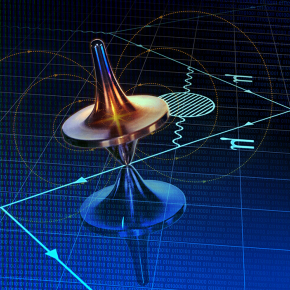For more than 10 years, the measurement of the magnetic properties of the muon has disagreed with theoretical predictions, suggesting a gap in the Standard Model of particle physics. On April 7, 2021, the first result of Fermilab's Muon g-2 experiment, which measures one of the properties of the muon, its magnetic moment, was unveiled.

A muon is about 200 times more massive than its electron cousin. Both act as if they have a tiny internal magnet (tied to their 1/2 spin). In a strong magnetic field, the direction of the muon magnet oscillates like the axis of a spinning top. The strength of the internal magnet determines the speed of precession of the muon in an external magnetic field: this "g factor" constitutes a high precision test of the Standard Model (with 11 significant digits after the decimal point).

A collaboration to which Michel Davier and Zhiqing Zhang (PHE PHE) belong played a decisive role in the calculation of the theoretical reference prediction. To determine the hadronic vacuum polarization contribution that limits the accuracy of the calculation, this collaboration used measurements from electron-positron colliders. The difference between this theoretical estimate and Fermilab's result is 4.2 sigmas: the probability that the results are a statistical fluctuation is about 1 in 40,000.

However, a different method has recently been followed by a European team of theoretical physicists, and it narrows the gap with experimental value. They calculated this contribution ab initio by numerically solving the Standard Model equations using the network QCD approach using several massively parallel European supercomputers. For the first time, such a calculation rivals in precision with the reference approach. To decide, we will have to wait until this theoretical calculation is confirmed by other teams and understand where the differences between the two approaches come from.

To know more: Fermilab website.